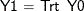# The MI Procedure

### Example 63.15 Creating Control-Based Pattern Imputation in Sensitivity Analysis

This example illustrates the pattern-mixture model approach to multiple imputation under the MNAR assumption by creating control-based pattern imputation.

Suppose that a pharmaceutical company is conducting a clinical trial to test the efficacy of a new drug. The trial consists of two groups of equally allocated patients: a treatment group that receives the new drug and a placebo control group. The variable `Trt` is an indicator variable, with a value of 1 for patients in the treatment group and a value of 0 for patients in the control group. The variable `Y0` is the baseline efficacy score, and the variable `Y1` is the efficacy score at a follow-up visit.

If the data set does not contain any missing values, then a regression model such ascan be used to test the the treatment effect.

Suppose that the variables `Trt` and `Y0` are fully observed and the variable `Y1` contains missing values in both the treatment and control groups. Multiple imputation for missing values often assumes that the values are missing at random. But if missing `Y1` values for individuals in the treatment group imply that these individuals no longer receive the treatment, then it is reasonable to assume that the conditional distribution of `Y1` given `Y0` for individuals who have missing `Y1` values in the treatment group is similar to the corresponding distribution of individuals in the control group.

Ratitch and O’Kelly (2011) describe an implementation of the pattern-mixture model approach that uses a control-based pattern imputation. That is, an imputation model for the missing observations in the treatment group is constructed not from the observed data in the treatment group but rather from the observed data in the control group. This model is also the imputation model that is used to impute missing observations in the control group.

Table 63.10 shows the variables in the data set. For the control-based pattern imputation, all missing `Y1` values are imputed based on the model that is constructed using observed `Y1` data from the control group (Trt=0) only.

Table 63.10: Variables

Variables

Trt

Y0

Y1

0

X

X

1

X

X

0

X

.

1

X

.

Suppose the data set `Mono1` contains the data from the trial that have missing values in `Y1`. Output 63.15.1 lists the first 10 observations.

Output 63.15.1: Clinical Trial Data

 First 10 Obs in the Trial Data

Obs Trt y0 y1
1 0 10.5212 11.3604
2 0 8.5871 8.5178
3 0 9.3274 .
4 0 9.7519 .
5 0 9.3495 9.4369
6 1 11.5192 13.2344
7 1 10.7841 .
8 1 9.7717 10.9407
9 1 10.1455 10.8279
10 1 8.2463 9.6844

The following statements implement the control-based pattern imputation:

```proc mi data=Mono1 seed=14823 nimpute=10 out=outex15;
class Trt;
monotone reg (/details);
mnar model( y1 / modelobs= (Trt='0'));
var y0 y1;
run;
```

The MNAR statement imputes missing values for scenarios under the MNAR assumption. The MODEL option specifies that only observations where TRT=0 are used to derive the imputation model for the variable `Y1`. Thus, `Y0` and `Y1` (but not Trt) are specified in the VAR list.

The "Model Information" table  in Output 63.15.2 describes the method that is used in the multiple imputation process.

Output 63.15.2: Model Information

The MI Procedure

Model Information
Data Set WORK.MONO1
Method Monotone
Number of Imputations 10
Seed for random number generator 14823

The "Monotone Model Specification"  table in Output 63.15.3 describes methods and imputed variables in the imputation model. The MI procedure uses the regression method to impute the variable `Y1`.

Output 63.15.3: Monotone Model Specification

Monotone Model Specification
Method Imputed
Variables
Regression y1

The "Missing Data Patterns"  table in Output 63.15.4 lists distinct missing data patterns and their corresponding frequencies and percentages. The table confirms a monotone missing pattern for these variables.

Output 63.15.4: Missing Data Patterns

Missing Data Patterns
Group y0 y1 Freq Percent Group Means
y0 y1
1 X X 75 75.00 9.996993 10.709706
2 X . 25 25.00 10.181488 .

By default, for each imputed variable, all available observations are used in the imputation model. When you specify the MODEL option in the MNAR statement, the "Observations Used for Imputation Models Under MNAR Assumption"  table in Output 63.15.5 lists the subset of observations that are used for the imputation model for `Y1`.

Output 63.15.5: Observations Used for Imputation Models under MNAR Assumption

Observations Used
for Imputation
Models Under MNAR
Assumption
Imputed
Variable
Observations
y1 Trt = 0

When you specify the DETAILS option, the parameters that are estimated from the observed data and the parameters that are used in each imputation are displayed in Output 63.15.6.

Output 63.15.6: Regression Model

Regression Models for Monotone Method
Imputed
Variable
Effect Obs-Data Imputation
1 2 3 4 5 6 7 8 9 10
y1 Intercept -0.30169 -0.174265 -0.280404 -0.275183 0.090601 -0.457480 -0.241909 -0.501351 -0.058460 -0.436650 -0.509949
y1 y0 0.69364 0.641733 0.629970 0.507776 0.752283 0.831001 0.970075 0.724584 0.623638 0.563499 0.621280

The following statements list the first 10 observations of the output data set `Outex15` in Output 63.15.7:

```proc print data=outex15(obs=10);
title 'First 10 Observations of the Imputed Data Set';
run;
```

Output 63.15.7: Imputed Data Set

 First 10 Observations of the Imputed Data Set

Obs _Imputation_ Trt y0 y1
1 1 0 10.5212 11.3604
2 1 0 8.5871 8.5178
3 1 0 9.3274 9.5786
4 1 0 9.7519 9.6060
5 1 0 9.3495 9.4369
6 1 1 11.5192 13.2344
7 1 1 10.7841 10.7873
8 1 1 9.7717 10.9407
9 1 1 10.1455 10.8279
10 1 1 8.2463 9.6844